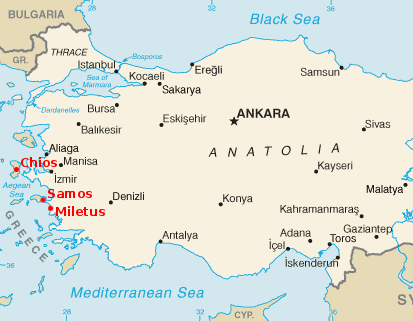#Thales of Miletus

Math Lair Home > Mathematicians > Thales of MiletusMap of modern Turkey, showing the location of ancient Miletus, the home of Thales.

Thales of Miletus lived from 634 B.C. to 548 B.C. In his youth, he travelled extensively. He likely visited Egypt and Babylon, two ancient civilisations which were still in existence. Later on, he founded the Ionian School, the first of its kind in Greece. Among others, Pythagoras would attend this famous school.

Thales is the first mathematician about whom we know something other than just the works he authored. While we have some ideas about Thales' accomplishments, both mathematical and non-mathematical, we cannot be sure that everything written about him is true. Some of his non-mathematical deeds were:

• He reportedly predicted a solar eclipse in Greece in 585 B.C., but this may not be true because of the primitive state of astronomy in Greece at the time.
• It was said that he made his fortune by buying all of the olive presses in Miletus and nearby Chios (see map at top of page for where these locations are), renting them out at harvest time at a high profit.
• He noticed that amber, when rubbed, attracted threads, feathers, and other light objects, thus discovering static electricity.

The later Greeks named Thales as the first of the seven wise men of Greece. He is the first person to have specific mathematical discoveries credited to him. These discoveries were:

• The diameter of a circle divides that circle into two parts of equal area.
• Two triangles are the same if they have two angles that are the same, and the lengths of the side between those two angles are the same.
• A triangle inscribed in a semicircle is a right triangle.
• An isosceles triangle has two equal angles.

You might think that everyone knows that, for example, a circle's diameter divides it into two, and that these discoveries are all obvious. Indeed they are. However, Thales' great advancement was that he was the first person to realize that he could prove his discoveries by means of logical deductions. This advancement was unique in human history; while other civilizations, such as those in China, India, and Mesoamerica, developed advanced mathematics, none of them made much use of deductive proof, a technique of great importance to modern mathematics.

Thales was also one of the first to look at the geometrical figures as abstractions rather than as real-world objects. While a line drawn in the sand may represent a line, it is not the line. A true "line" is an abstract concept of a geometrical figure that is infinitely long, infinitely thin, and perfectly straight, something that a line drawn in (for example) the sand could never be. These features of Thales' work are a step forward from all previous mathematical work, such as that of the Egyptians and Babylonians.

Thales is also credited with practical mathematical achievements as well. As an example, he is said to have measured the height of the Great Pyramid of Cheops. The method used is straightforward and is one that you can use today to measure the heights of tall objects: He showed that the right triangle whose two legs consisted of a man and his shadow was similar to the right triangle whose two legs were the height of the pyramid and its shadow at the same time of the day. Since the ratio between the two sides of both triangles are in proportion, the height of the pyramid can be found by:

height of pyramid = (height of man / length of man's shadow) × length of pyramid's shadow